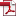• KSII Transactions on Internet and Information Systems
Monthly Online Journal (eISSN: 1976-7277)

# ASSVD: Adaptive Sparse Singular Value Decomposition for High Dimensional Matrices

Vol. 14, No. 6, June 30, 202010.3837/tiis.2020.06.017, Download Paper (Free):#### Abstract

In this paper, an adaptive sparse singular value decomposition (ASSVD) algorithm is proposed to estimate the signal matrix when only one data matrix is observed and there is high dimensional white noise, in which we assume that the signal matrix is low-rank and has sparse singular vectors, i.e. it is a simultaneously low-rank and sparse matrix. It is a structured matrix since the non-zero entries are confined on some small blocks. The proposed algorithm estimates the singular values and vectors separable by exploring the structure of singular vectors, in which the recent developments in Random Matrix Theory known as anisotropic Marchenko-Pastur law are used. And then we prove that when the signal is strong in the sense that the signal to noise ratio is above some threshold, our estimator is consistent and outperforms over many state-of-the-art algorithms. Moreover, our estimator is adaptive to the data set and does not require the variance of the noise to be known or estimated. Numerical simulations indicate that ASSVD still works well when the signal matrix is not very sparse.